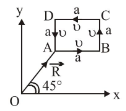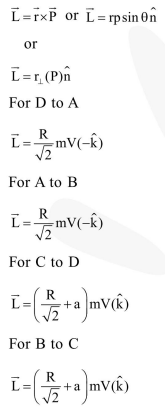Deepak Scored 45->99%ile with Bounce Back Crack Course. You can do it too!

# A particle of mass

Question:

A particle of mass $m$ is moving along the side of a square of side ' $a$ ', with a uniform speed $v$ in the $x$-y plane as shown in the figure :Which of the following statement is false for the angular momentum $\overrightarrow{\mathrm{L}}$ about the origin ?

1. $\overrightarrow{\mathrm{L}}=\frac{\mathrm{mv}}{\sqrt{2}} \mathrm{R} \hat{\mathrm{k}}$ when the particle is moving from $\mathrm{D}$ to $\mathrm{A}$

2. $\overrightarrow{\mathrm{L}}=-\frac{\mathrm{mv}}{\sqrt{2}} \mathrm{R} \hat{\mathrm{k}}$ when the particle is moving from $\mathrm{A}$ to $\mathrm{B}$

3. $\overrightarrow{\mathrm{L}}=m v\left[\frac{\mathrm{R}}{\sqrt{2}}-\mathrm{a}\right] \hat{\mathrm{k}}$ when the particle is moving from $\mathrm{C}$ to $\mathrm{D}$

4. $\overrightarrow{\mathrm{L}}=\mathrm{mv}\left[\frac{\mathrm{R}}{\sqrt{2}}+\mathrm{a}\right] \hat{\mathrm{k}}$ when the particle is moving from $\mathrm{B}$ to $\mathrm{C}$

Correct Option: 1, 3

Solution: